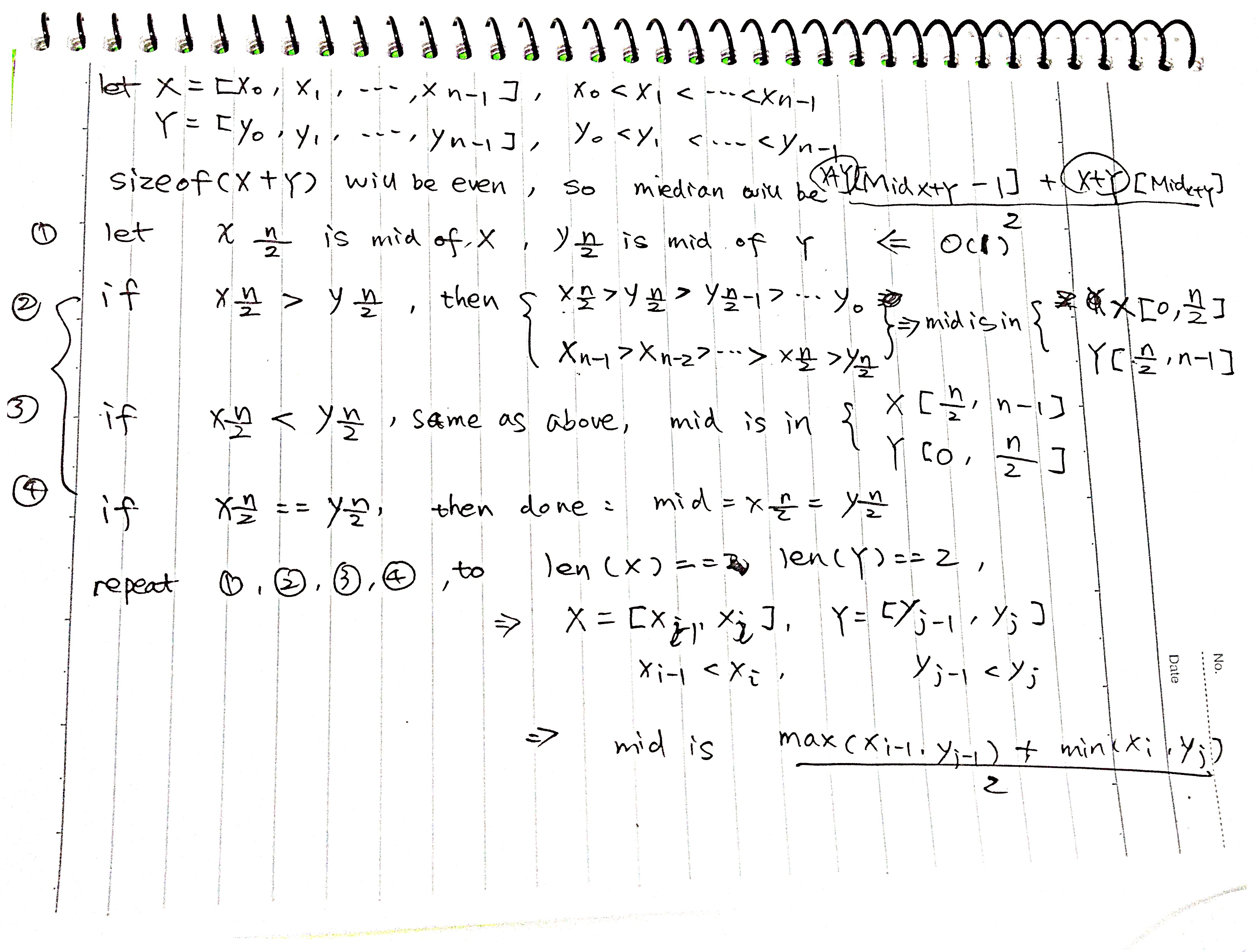Question: There are 2 sorted arrays X and Y of size n each. Write an algorithm to find the median of the array obtained after merging the above 2 arrays(i.e. array of length 2n). The complexity should be O(log(n))

### Formal Deduction